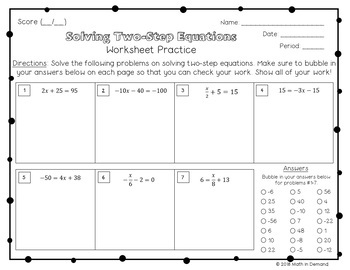Total:
\$0.00# 7th Grade Math Practice Worksheets DISTANCE LEARNING7th
Subjects
Resource Type
Formats Included
• Zip
Pages

#### Also included in

1. ⭐ This bundle includes ALL of my 7th Grade Math material in my store and ALL future 7th Grade Math Products ⭐This includes all of my popular 7th grade math interactive notebooks, practice worksheets, foldables, activities, warm-ups, homework, exit slips, assessments, early finishers, and SO MUCH MOR
\$249.99
\$463.89
Save \$213.90
2. ⭐ This bundle includes ALL of my Math Curriculum in my Teachers Pay Teachers store ⭐You will be receiving ALL of my 6th, 7th, and 8th Grade Math interactive notebooks, activities, foldables, practice worksheets, warm-ups, exit slips, assessments, and much more! This is well over 1000 pages of materi
\$899.99
\$1718.65
Save \$818.66
3. This listing is for a whole years worth of practice worksheets for 6th, 7th, and 8th grade math (some worksheets are still in progress). That is 163 math worksheets when all worksheets are completed!!! These worksheets are self assessing because they include a bubble-in area for the answer key. Som
\$74.99
\$119.97
Save \$44.98

### Description

This includes a whole years worth of practice worksheets for 7th grade math. These worksheets are self-assessing because they include a bubble-in area for the answer key. There are over 60 worksheets! I have broken up my worksheets into 5 units. These 5 units are:

Unit 1 - Number Sense

Unit 2 - Ratios & Proportional Relationships

Unit 3 - Expressions & Equations

Unit 4 - Geometry

Unit 5 - Statistics & Probability

A breakdown of each unit is below. There will be a worksheet for every concept below:

Unit 1 - Number Sense

-Absolute Value

-Numbers on the Number Line

-Adding & Subtracting Integers on the Number Line

-Adding & Subtracting Fractions on the Number Line

-Adding & Subtracting Integers using Visual Representation

-Adding & Subtracting Integers using Rules

-Multiplying Integers

-Dividing Integers

-Least Common Multiple & Greatest Common Factor

-Multiplying Fractions

-Dividing Fractions

-Multiplying Decimals

-Dividing Decimals

Unit 2 - Ratios & Proportional Relationships

-Intro to Ratios

-Intro to Rates

-Unit Rates Word Problems

-Writing Proportions

-Solving Proportions

-Proportional Relationships Equation

-Proportional Relationship in a Table and Graph

-Unit Rate on a Graph

-Converting between Decimals, Fractions, and Percents

-Markdowns/Discounts

-Markups

-Sales Tax

-Tip

-Commission

-Percent Increase/Decrease

-Simple Interest

Unit 3 - Expressions & Equations

-Combining Like Terms

-Distributive Property

-Simplifying Expressions

-Solving One-Step Equations

-Solving Two-Step Equations with Modeling

-Solving Two-Step Equations

-Solving and Graphing One-Step Inequalities

-Solving and Graphing Two-Step Inequalities

Unit 4 - Geometry

-Constructing Triangles

-Intro to Angle Relationships

-Evaluating Angles

-Identifying Angle Relationships

-Solving with Angle Relationships

-Rectangles: Area and Perimeter

-Triangles: Area and Perimeter

-Circles: Area and Circumference

-Similar Figures

-Scale Drawings

-Volume

-Surface Area using Nets

Unit 5 - Statistics & Probability

-Random Sampling

-Draw Inferences

-Numerical Data Distributions

-Mean, Median, Mode, and Range

-Mean Absolute Deviation

-Line Plots

-Stem and Leaf Plot

-Box and Whisker Plot

-Probability of an Event

-Probability of a Repeated Event

-Probability of Independent and Dependent Events

-Likelihood

Total Pages
Included
Teaching Duration
1 Year
Report this Resource to TpT
Reported resources will be reviewed by our team. Report this resource to let us know if this resource violates TpT’s content guidelines.